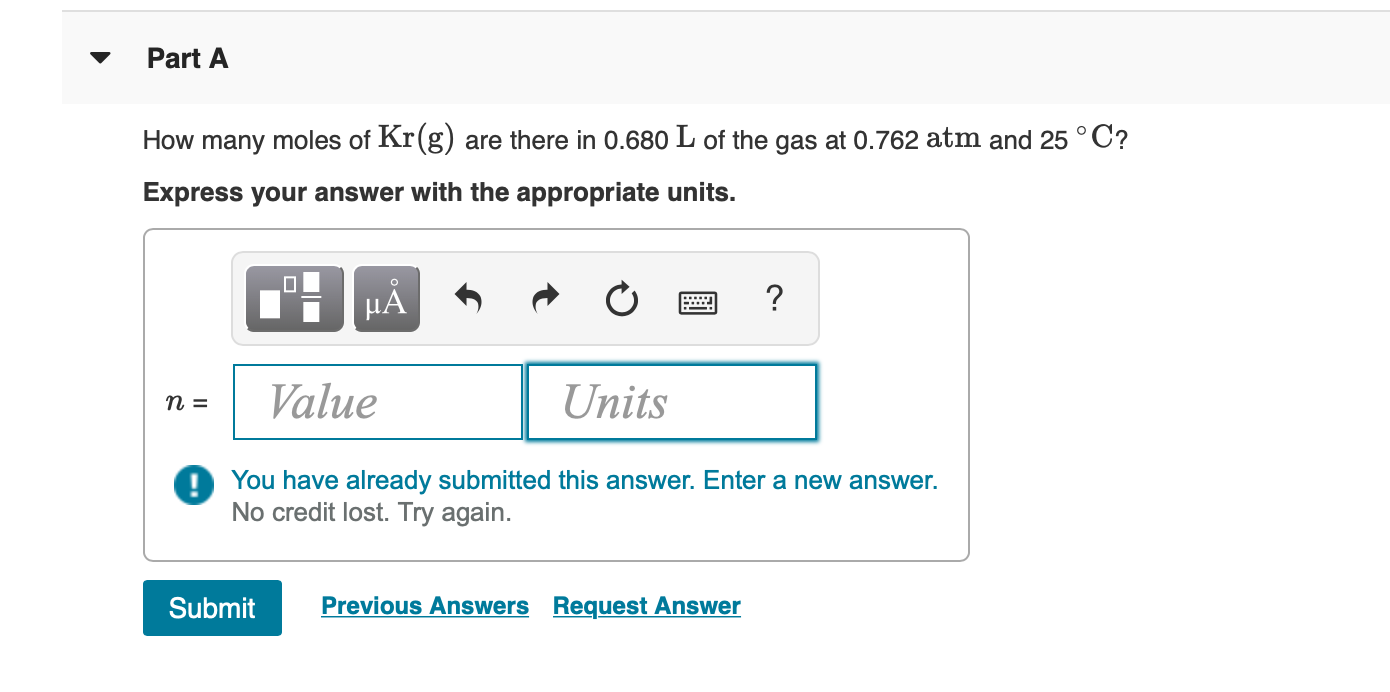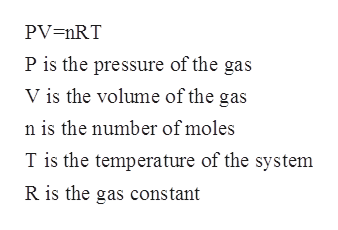# Part AHow many moles of Kr(g) are there in 0.680 L of the gas at 0.762 atm and 25 °C?Express your answer with the appropriate units.?НА3D иValueUnitsDYou have already submitted this answer. Enter a new answer.No credit lost. Try again.Previous Answers Request AnswerSubmit

Question
86 views

I can't seem to solve this..help_outlineImage TranscriptionclosePart A How many moles of Kr(g) are there in 0.680 L of the gas at 0.762 atm and 25 °C? Express your answer with the appropriate units. ? НА 3D и Value Units DYou have already submitted this answer. Enter a new answer. No credit lost. Try again. Previous Answers Request Answer Submit fullscreen
check_circle

Step 1

For a given chemical species, the number of moles can be defined to the ratio of mass to the molar mass. The mathematical formula which is used to calculate the number of moles is as follows:

Step 2

The ideal gas law that is also known as the equation of state is an equation of state of a hypothetical ideal gas. The equation can be written as shown below:help_outlineImage TranscriptionclosePV=nRT P is the pressure of the gas V is the volume of the gas n is the number of moles T is the temperature of the system R is the gas constant fullscreen
Step 3

The volume of the Kr in the gas is given to be 0.680 L.

T=25oC=298 K

P= 0.762 at...

### Want to see the full answer?

See Solution

#### Want to see this answer and more?

Solutions are written by subject experts who are available 24/7. Questions are typically answered within 1 hour.*

See Solution
*Response times may vary by subject and question.
Tagged in

### Physical Chemistry